Section 1
Section 2
Section 3
Section 4
Section 5
Section 6
Section 7
Section 8
Section 9
Section 10
Section 11
###### Object Oriented Python
Introduction to Python

# Introduction to Python Programming language

Python is developed by Guido van Rossum. Guido van Rossum started implementing Python in 1989. Python is a very simple programming language so even if you are new to programming, you can learn python without facing any issues.

Interesting fact: Python is named after the comedy television show Monty Python’s Flying Circus. It is not named after the Python snake.

## Features of Python programming language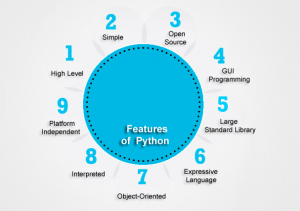1. High Level Language:  Python is a high level programming language.

2. Simple: Learning python is easy as this is a expressive and high level programming language, which means it is easy to understand the language and thus easy to learn.

3. Open Source: Python is an open source programming language.

4. GUI Programming: You can design Graphical user interfaces using Python.

5. Large standard library: Python comes with a large standard library that has some handy codes and functions which we can use while writing code in Python.

6. Expressive Language: Python language is more expressive. The sense of expressive is the code is easily understandable.

7. Object-Oriented language: It is Object-Oriented programming language. It follow object and class concept.

8. Interpreted: Python code is interpreted by interpreter line by line at a time.

9. Platform Independent: It is platform independent programming language, its code easily run on any platform such as Windows, Linux, Unix , Macintosh etc. Thus, Python is a portable language.

Other features of Python includes:

Automatic memory management: Python supports automatic memory management which means the memory is cleared and freed automatically. You do not have to bother clearing the memory.

Supports exception handling: If you are new, you may wonder what is an exception? An exception is an event that can occur during program exception and can disrupt the normal flow of program. Python supports exception handling which means we can write less error prone code and can test various scenarios that can cause an exception later on.

Free: Python is free to download and use. This means you can download it for free and use it in your application. See: Open Source Python License. Python is an example of a FLOSS (Free/Libre Open Source Software), which means you can freely distribute copies of this software, read its source code and modify it.

Cross platform: Python is available and can run on various operating systems such as Mac, Windows, Linux, Unix etc. This makes it a cross platform and portable language.

## What Can You Do with Python?

You may be wondering what all are the applications of Python. There are so many applications of Python, here are some of the them.
1. Web development – Web framework like Django and Flask are based on Python. They help you write server side code which helps you manage database, write backend programming logic, mapping urls etc.

2. Machine learning – There are many machine learning applications written in Python. Machine learning is a way to write a logic so that a machine can learn and solve a particular problem on its own. For example, products recommendation in websites like Amazon, Flipkart, eBay etc. is a machine learning algorithm that recognises user’s interest. Face recognition and Voice recognition in your phone is another example of machine learning.

3. Data Analysis – Data analysis and data visualisation in form of charts can also be developed using Python.

4. Scripting – Scripting is writing small programs to automate simple tasks such as sending automated response emails etc. Such type of applications can also be written in Python programming language.

5. Game development – You can develop games using Python.

6. You can develop Embedded applications in Python.

7. Desktop applications – You can develop desktop application in Python using library like TKinter or QT.

## How to install Python

Python installation is pretty simple, you can install it on any operating system such as Windows, Mac OS X, Ubuntu etc. Just follow the steps in this article – Python Installation

# How to Install Python

You can install Python on any operating system such as Windows, Mac OS X, Linux/Unix and others.

This is the official Python website and it will detect the operating system and based on that it would recommend you to download Python. Here I am using Mac OS X so it gave me the download options for Python 2 and Python 3 for Mac OS X. I would recommend you to download the latest version of Python 3 (Python 3.6.4 in the screenshot).

Installation steps are pretty simple. You just have to accept the agreement and finish the installation.

If you are using Mac OS X then you may notice that Python is already installed in your system,
however it would most likely be a Python 2 so you can download the latest Python 3 version from here and install it in your system.

# Install PyCharm Python IDE in Windows, Mac OS X, Linux/Unix

In the previous tutorials we have seen the Python introduction and installation. In this guide, we will learn how to install PyCharm (a popular IDE for Python programming).

If you are new to programming then you may be wondering what do we mean by IDE? IDE stands for integrated development environment. It is a software that consolidates the basic tools that are required to write and test programs in a certain language. Typically, an IDE contains a code editor, a compiler or interpreter and a debugger that you can access at the same place through IDE GUI.

## PyCharm Installation

Mac: Double click the .dmg file and drag PyCharm to the Application Folder.
Windows: Double click the .exe file and follow the installation steps for the default PyCharm installation.

## Launch PyCharm

Mac: Go to the Applications folder and click on the PyCharm icon. Alternatively, you can drag the icon to your dock to open the IDE quickly by clicking on the icon in dock.

Windows: If you have followed the default installation process then you can see the PyCharm icon on your desktop. If you don’t find the icon then go to the PyCharm folder – C:\Program Files (x86)\JetBrains\PyCharm 2017.1\bin (the path may be different for your system) and click on the PyCharm.exe file to launch the IDE

#### Whats Next

We will create a Python project in PyCharm IDE in the next tutorial.

# First Python Project in PyCharm IDE

In the last tutorial we have seen how to install PyCharm (a popular IDE for Python). In this guide, we will see how to create your First Python project in PyCharm.## Creating Python Project in PyCharm

1. Click “Create New Project” in the PyCharm welcome screen.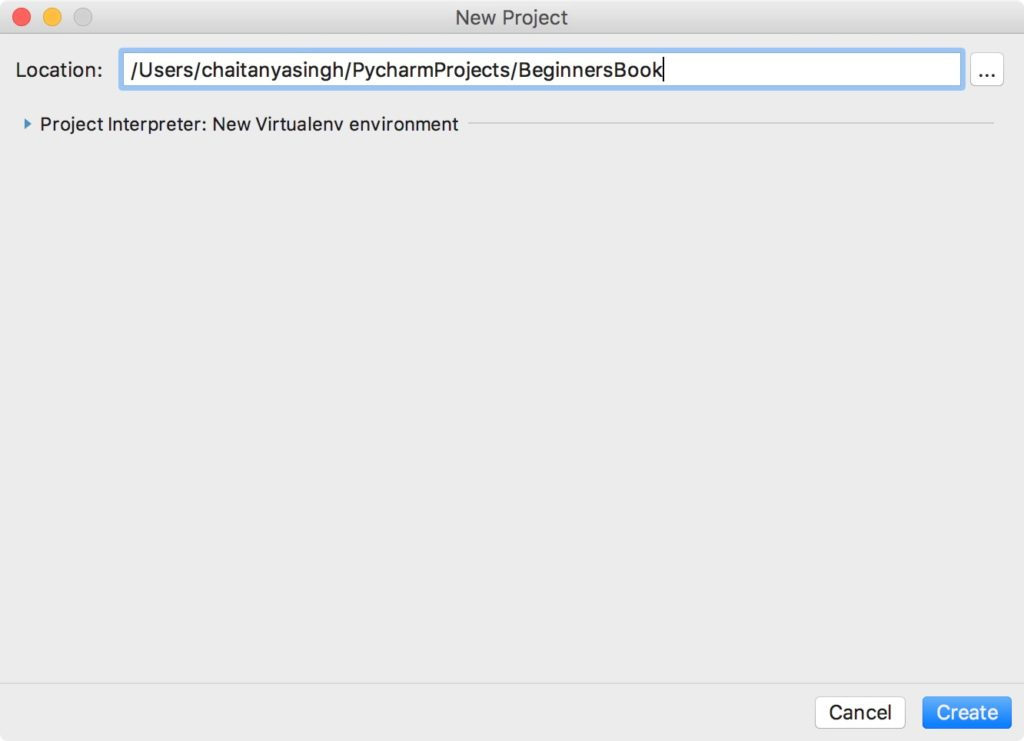## Writing and running your first Python Program

1. Now that we have created a Python project, it’s time to create a Python program file to write and run our first Python program. To create a file, right click on the folder name > New > Python File (as shown in the screenshot). Give the file name as “HelloWorld” and click ok.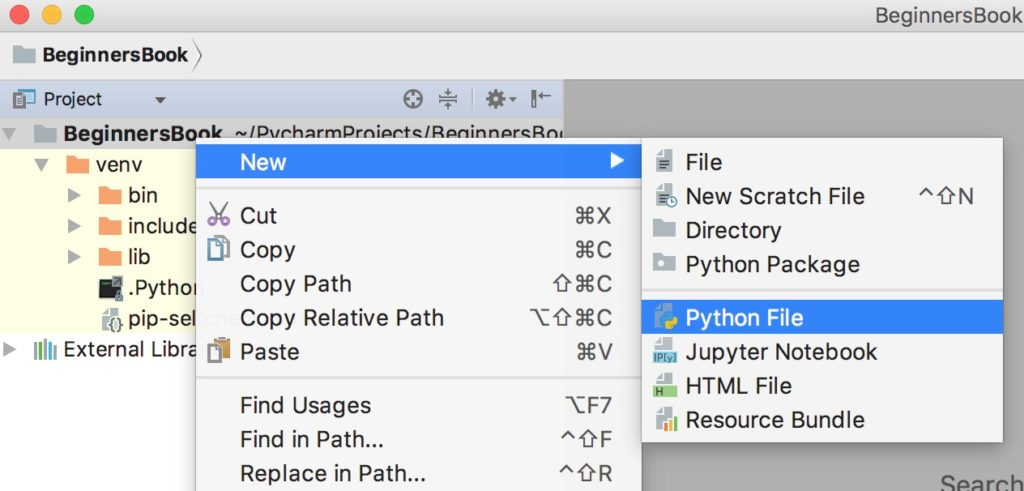2. Write the following code in the file.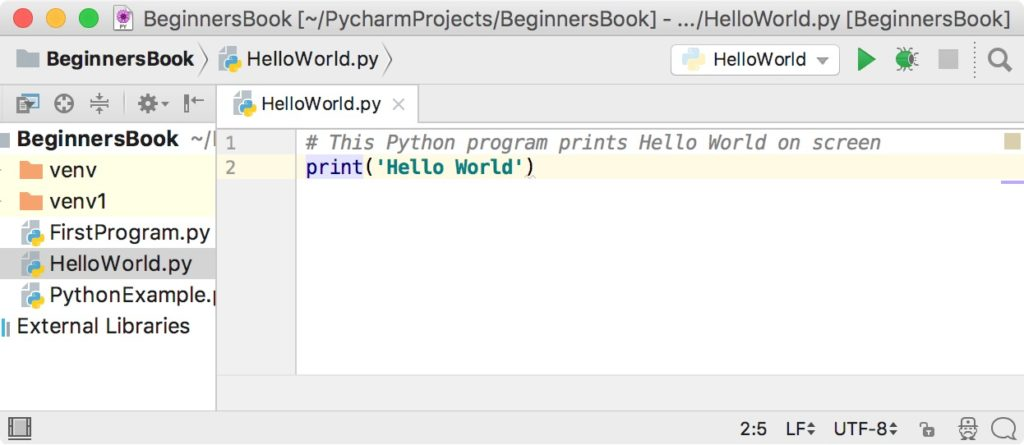3. Lets run the code. Right click on the HelloWorld.py file (or the name you have given while creating Python file) in the left sidebar and click on ‘Run HelloWorld’.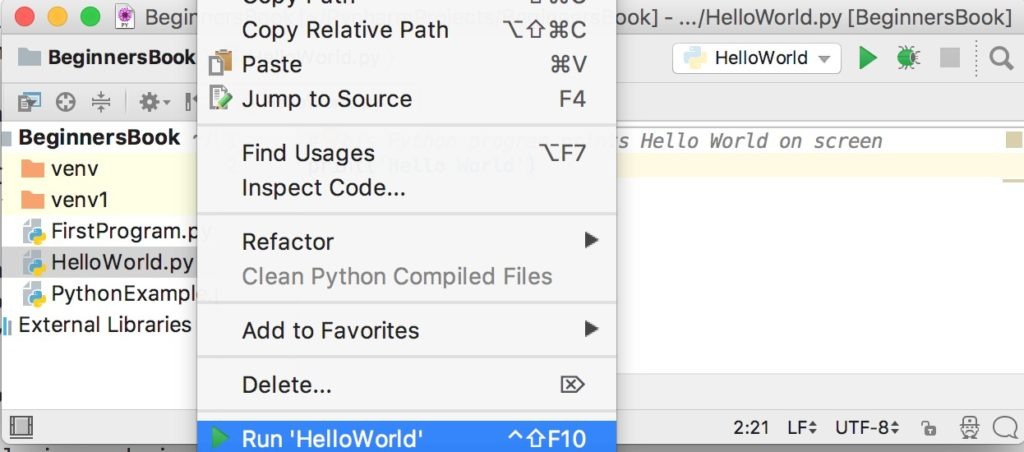4. You can see the output of the program at the bottom of the screen.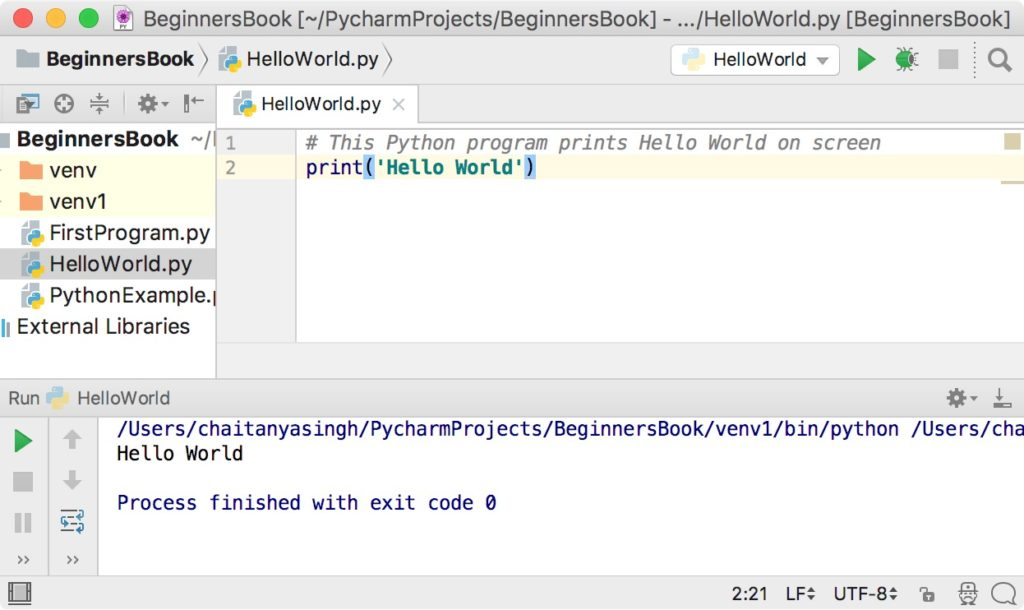Although comments do not change the outcome of a program, they still play an important role in any programming and not just Python. Comments are the way to improve the readability of a code, by explaining what we have done in code in simple English. In this guide, we will learn about comments in Python and their types.

Before we go through an example of comments in Python. Lets first understand the need of comments in Python or in any programming language.

A comment is text that doesn’t affect the outcome of a code, it is just a piece of text to let someone know what you have done in a program or what is being done in a block of code. This is especially helpful when someone else has written a code and you are analysing it for bug fixing or making a change in logic, by reading a comment you can understand the purpose of code much faster then by just going through the actual code.

Lets see how a comment looks like in Python.

```# This is just a text, it won't be executed.

print("Python comment example")```

Output: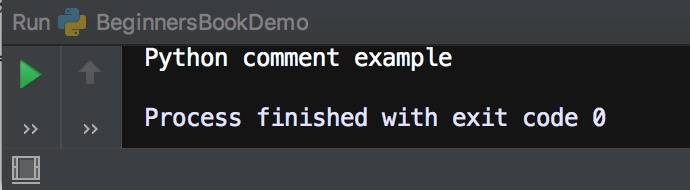## Types of Comments in Python

There are two types of comments in Python.
1. Single line comment
2. Multiple line comment

## Single line comment

In python we use # special character to start the comment. Lets take few examples to understand the usage.

`# This is just a comment. Anything written here is ignored by Python`

## Multi-line comment:

To have a multi-line comment in Python, we use triple single quotes at the beginning and at the end of the comment, as shown below.

```'''
This is a
multi-line
comment
'''```

In this Python program we are seeing three types of comments. Single line comment, multi-line comment and the comment that is starting in the same line after the code.

```'''
We are writing a simple program here
First print statement.
This is a multiple line comment.
'''
print("Hello Guys")

# Second print statement
print("How are You all?")

print("Welcome to BeginnersBook") # Third print statement```

Output: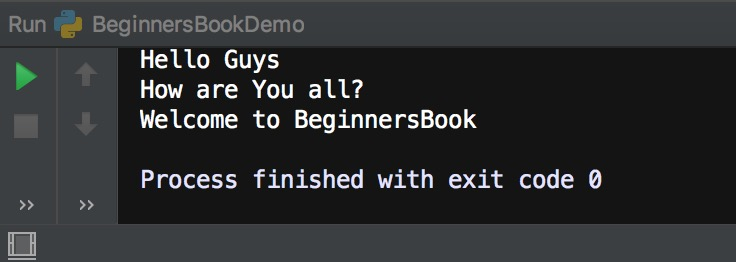## # character inside quotes

When # character is encountered inside quotes, it is not considered as comment. For example:

`print("#this is not a comment")`

Output:

## #this is not a comment

Python Variables with examples

Variables are used to store data, they take memory space based on the type of value we assigning to them. Creating variables in Python is simple, you just have write the variable name on the left side of = and the value on the right side, as shown below. You do not have to explicitly mention the type of the variable, python infer the type based on the value we are assigning.
```num = 100      #num is of type int
str = "Chaitanya"      #str is of type string```

## Variable name – Identifiers

Variable name is known as identifier. There are few rules that you have to follow while naming the variables in Python.
1. The name of the variable must always start with either a letter or an underscore (_). For example: _str, str, num, _num are all valid name for the variables.
2. The name of the variable cannot start with a number. For example: 9num is not a valid variable name.
3. The name of the variable cannot have special characters such as %, \$, # etc, they can only have alphanumeric characters and underscore (A to Z, a to z, 0-9 or _ ).
4. Variable name is case sensitive in Python which means `num` and `NUM` are two different variables in python.

## Python Variable Example

```num = 100
str = "BeginnersBook"
print(num)
print(str)```

Output: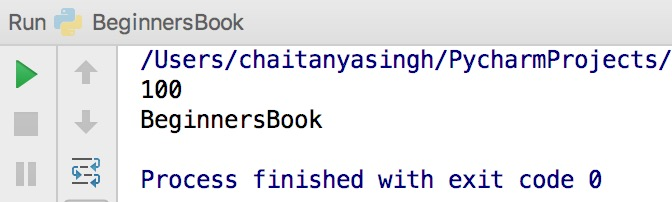## Python multiple assignment

We can assign multiple variables in a single statement like this in Python.

```x = y = z = 99
print(x)
print(y)
print(z)```

Output:

```99
99
99```

Another example of multiple assignment

```a, b, c = 5, 6, 7
print(a)
print(b)
print(c)```

Output: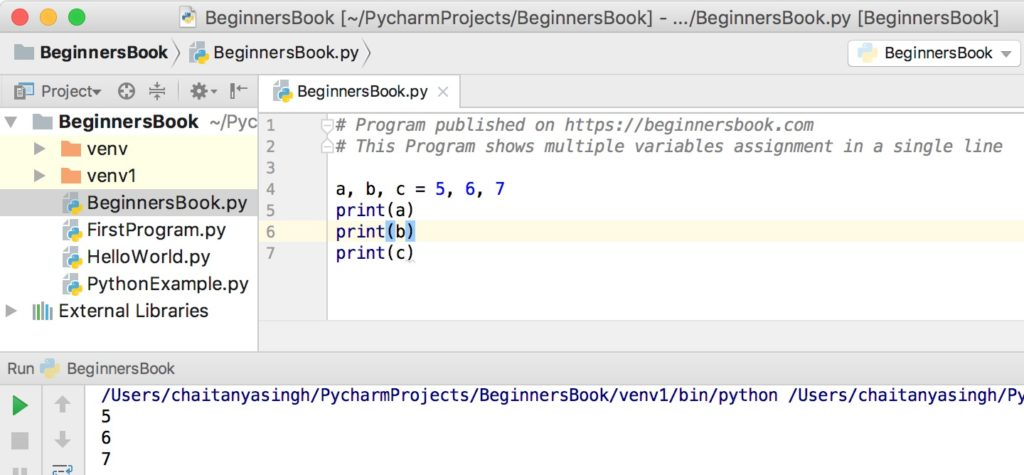## Plus and concatenation operation on the variables

```x = 10
y = 20
print(x + y)

p = "Hello"
q = "World"
print(p + " " + q)```

Output: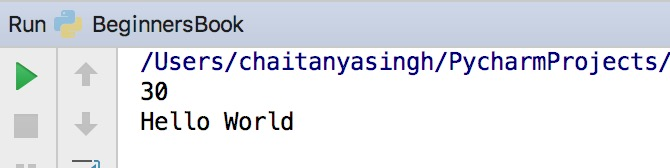However if you try to use the + operator with variable `x` and `p` then you will get the following error.

`unsupported operand type(s) for +: 'int' and 'str'`

## Data Types

A data type defines the type of data, for example 123 is an integer data while “hello” is a String type of data. The data types in Python are divided in two categories:
1. Immutable data types – Values cannot be changed.
2. Mutable data types – Values can be changed

Immutable data types in Python are:
1. Numbers
2. String
3. Tuple

Mutable data types in Python are:
1. List
2. Dictionaries
3. Sets

# Python Keywords and Identifiers with examples

In this article, we will discuss Keywords and Identifiers in Python with the help of examples.

## What is a Python Keyword?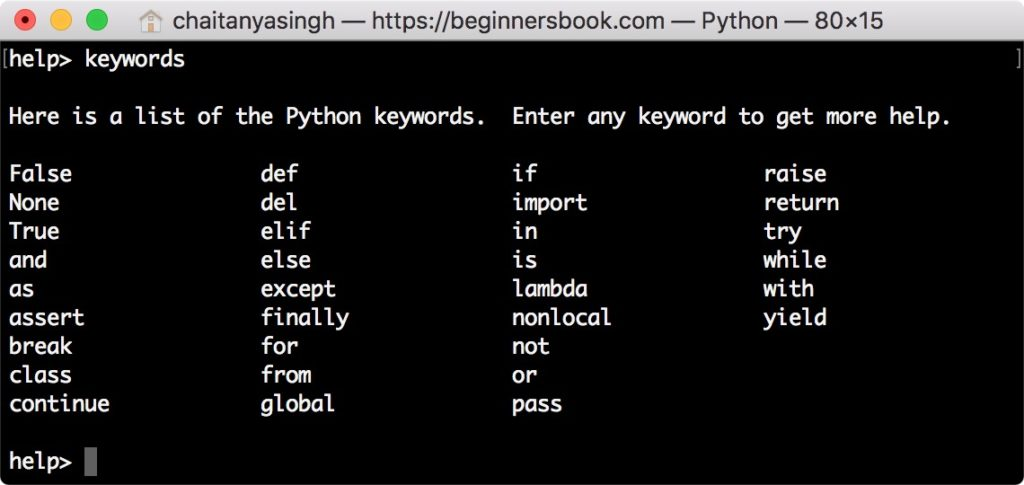A python keyword is a reserved word which you can’t use as a name of your variable, class, function etc. These keywords have a special meaning and they are used for special purposes in Python programming language. For example – Python keyword “while” is used for while loop thus you can’t name a variable with the name “while” else it may cause compilation error.

There are total 33 keywords in Python 3.6. To get the keywords list on your operating system, open command prompt (terminal on Mac OS) and type “Python” and hit enter. After that type `help()` and hit enter. Type `keywords` to get the list of the keywords for the current python version running on your operating system.

```Chaitanyas-MacBook-Pro:~ chaitanyasingh\$ Python 3.6
...
>>> help()
Welcome to Python 3.6's help utility!
...
help> keywords

Here is a list of the Python keywords.  Enter any keyword to get more help.

False               def                 if                  raise
None                del                 import              return
True                elif                in                  try
and                 else                is                  while
as                  except              lambda              with
assert              finally             nonlocal            yield
break               for                 not
class               from                or
continue            global              pass```

## Example of Python keyword

In the following example we are using while keyword to create a loop for displaying the values of variables num as long as they are greater than 5.

```num = 10
while num>5:
print(num)
num -= 1```

Output: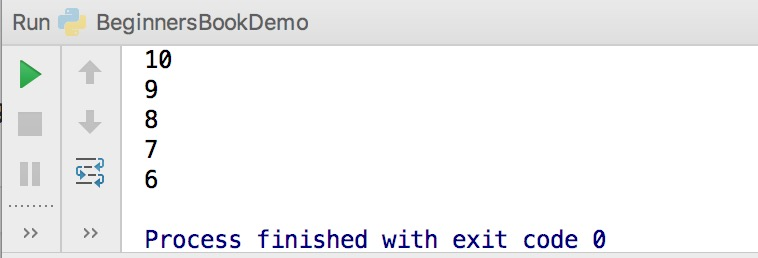## Python Identifiers

In the last article, we discussed about variables in Python.

Variable name is known as identifier. There are few rules that you have to follow while naming the variables in Python.

For example here the variable is of integer type that holds the value 10. The name of the variable, which is `num` is called identifier.

`num = 10`

1. The name of the variable must always start with either a letter or an underscore (_). For example: _str, str, num, _num are all valid name for the variables.
2. The name of the variable cannot start with a number. For example: 9num is not a valid variable name.
3. The name of the variable cannot have special characters such as %, \$, # etc, they can only have alphanumeric characters and underscore (A to Z, a to z, 0-9 or _ ).
4. Variable name is case sensitive in Python which means `num` and `NUM` are two different variables in python.

## Python identifier example

In the following example we have three variables. The name of the variables `num``_x` and `a_b` are the identifiers.

```# few examples of identifiers

num = 10
print(num)

_x = 100
print(_x)

a_b = 99
print(a_b)```

Output: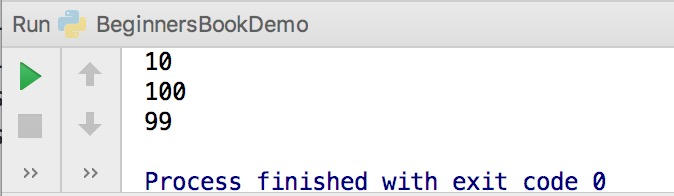# Python Data Types

Data type defines the type of the variable, whether it is an integer variable, string variable, tuple, dictionary, list etc. In this guide, you will learn about the data types and their usage in Python.

## Python data types

Python data types are divided in two categories, mutable data types and immutable data types.

Immutable Data types in Python
1. Numeric
2. String
3. Tuple

Mutable Data types in Python
1. List
2. Dictionary
3. Set

## 1. Numeric Data Type in Python

Integer – In Python 3, there is no upper bound on the integer number which means we can have the value as large as our system memory allows.

```# Integer number
num = 100
print(num)
print("Data Type of variable num is", type(num))```

Output: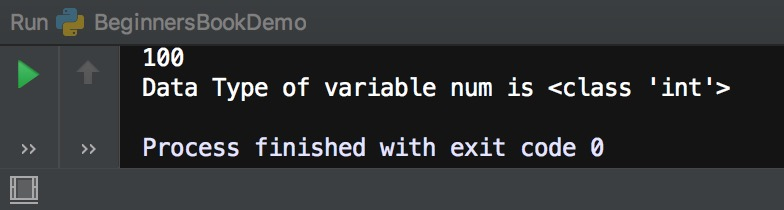Long – Long data type is deprecated in Python 3 because there is no need for it, since the integer has no upper limit, there is no point in having a data type that allows larger upper limit than integers.

Float – Values with decimal points are the float values, there is no need to specify the data type in Python. It is automatically inferred based on the value we are assigning to a variable. For example here fnum is a float data type.

```# float number
fnum = 34.45
print(fnum)
print("Data Type of variable fnum is", type(fnum))```

Output: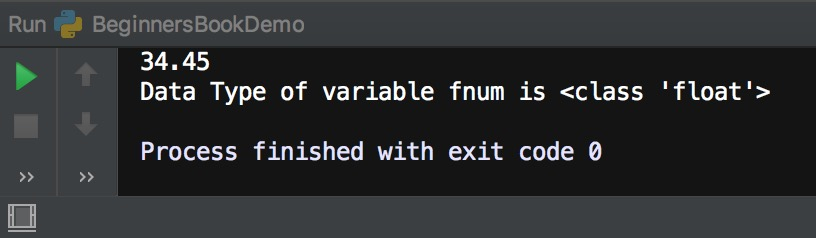Complex Number – Numbers with real and imaginary parts are known as complex numbers. Unlike other programming language such as Java, Python is able to identify these complex numbers with the values. In the following example when we print the type of the variable cnum, it prints as complex number.

```# complex number
cnum = 3 + 4j
print(cnum)
print("Data Type of variable cnum is", type(cnum))```

Output: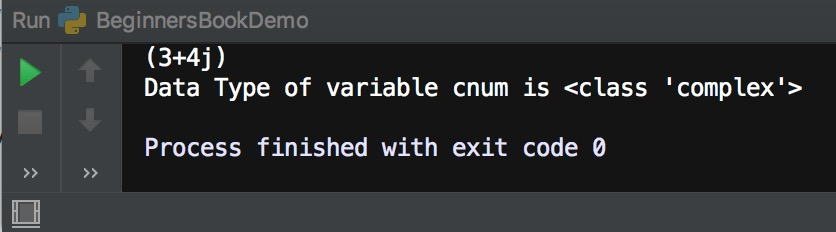## Binary, Octal and Hexadecimal numbers

In Python we can print decimal equivalent of binary, octal and hexadecimal numbers using the prefixes.
0b(zero + ‘b’) and 0B(zero + ‘B’) – Binary Number
0o(zero + ‘o’) and 0O(zero + ‘O’) – Octal Number
0x(zero + ‘x’) and 0X(zero + ‘X’) – Hexadecimal Number

```# integer equivalent of binary number 101
num = 0b101
print(num)

# integer equivalent of Octal number 32
num2 = 0o32
print(num2)

# integer equivalent of Hexadecimal number FF
num3 = 0xFF
print(num3)```

Output: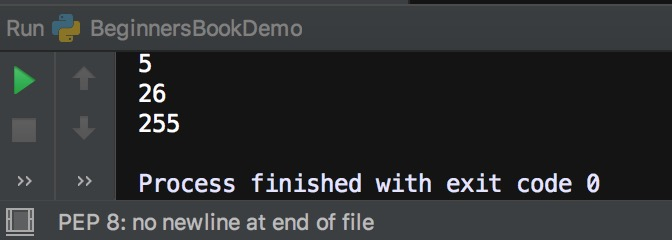## 2. Python Data Type – String

String is a sequence of characters in Python. The data type of String in Python is called “str”.

Strings in Python are either enclosed with single quotes or double quotes. In the following example we have demonstrated two strings one with the double quotes and other string s2 with the single quotes. To read more about strings, refer this article: Python Strings.

```# Python program to print strings and type

s = "This is a String"
s2 = 'This is also a String'

# displaying string s and its type
print(s)
print(type(s))

# displaying string s2 and its type
print(s2)
print(type(s2))```

Output: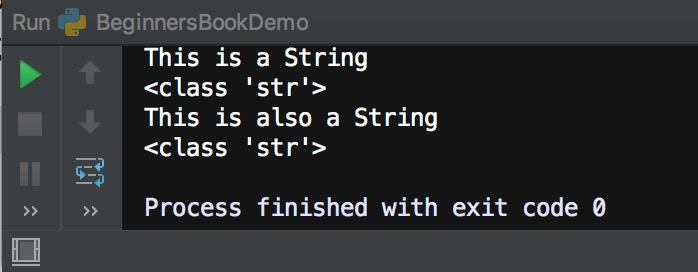## 3. Python Data Type – Tuple

Tuple is immutable data type in Python which means it cannot be changed. It is an ordered collection of elements enclosed in round brackets and separated by commas. To read more about tuple, refer this tutorial: Python tuple.

```# tuple of integers
t1 = (1, 2, 3, 4, 5)
# prints entire tuple
print(t1)

# tuple of strings
t2 = ("hi", "hello", "bye")
# loop through tuple elements
for s in t2:
print (s)

# tuple of mixed type elements
t3 = (2, "Lucy", 45, "Steve")
'''
Print a specific element
'''
print(t3)```

Output: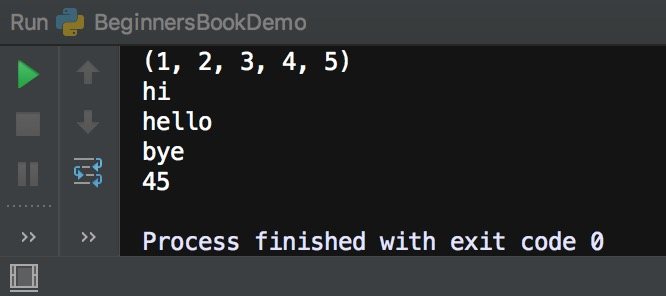## 4. Python Data Type – List

List is similar to tuple, it is also an ordered collection of elements, however list is a mutable data type which means it can be changed unlike tuple which is an immutable data type.

A list is enclosed with square brackets and elements are separated by commas. To read more about Lists, refer this guide: Python Lists

```# list of integers
lis1 = [1, 2, 3, 4, 5]
# prints entire list
print(lis1)

# list of strings
lis2 = ["Apple", "Orange", "Banana"]
# loop through list elements
for x in lis2:
print (x)

# List of mixed type elements
lis3 = [20, "Chaitanya", 15, "BeginnersBook"]
'''
Print a specific element in list
'''
print("Element at index 3 is:",lis3)```

Output:## 5. Python Data Type – Dictionary

Dictionary is a collection of key and value pairs. A dictionary doesn’t allow duplicate keys but the values can be duplicate. It is an ordered, indexed and mutable collection of elements. To read more about it refer: Python dictionary.

The keys in a dictionary doesn’t necessarily to be a single data type, as you can see in the following example that we have 1 integer key and two string keys.

```# Dictionary example

dict = {1:"Chaitanya","lastname":"Singh", "age":31}

# prints the value where key value is 1
print(dict)
# prints the value where key value is "lastname"
print(dict["lastname"])
# prints the value where key value is "age"
print(dict["age"])```

Output: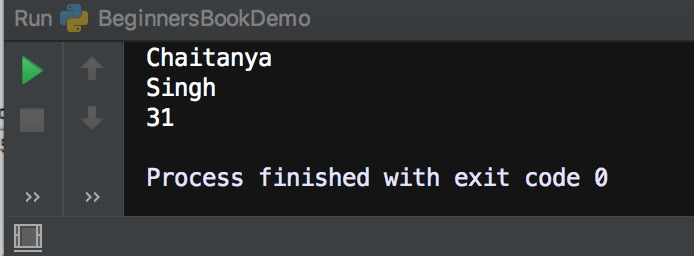## 6. Python Data Type – Set

A set is an unordered and unindexed collection of items. This means when we print the elements of a set they will appear in the random order and we cannot access the elements of set based on indexes because it is unindexed.

Elements of set are separated by commas and enclosed in curly braces. Lets take an example to understand the sets in Python.

```# Set Example
myset = {"hi", 2, "bye", "Hello World"}

# loop through set
for a in myset:
print(a)

# checking whether 2 exists in myset
print(2 in myset)

print(myset)```

Output: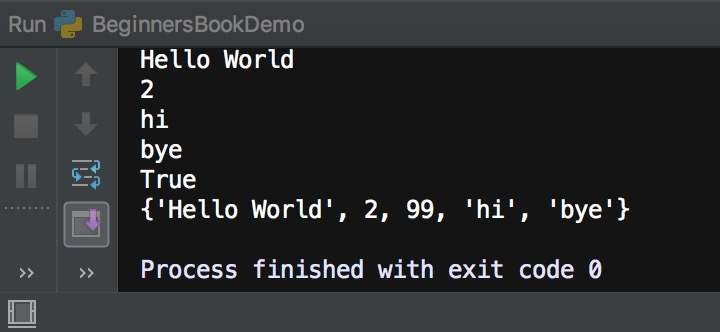# Python If Statement explained with examples

If statements are control flow statements which helps us to run a particular code only when a certain condition is satisfied. For example, you want to print a message on the screen only when a condition is true then you can use if statement to accomplish this in programming. In this guide, we will learn how to use if statements in Python programming with the help of examples.

There are other control flow statements available in Python such as if..else, if..elif..else,
nested if etc. However in this guide, we will only cover the if statements, other control statements are covered in separate tutorials.

## Syntax of If statement in Python

The syntax of if statement in Python is pretty simple.

```if condition:
block_of_code```

## If statement flow diagram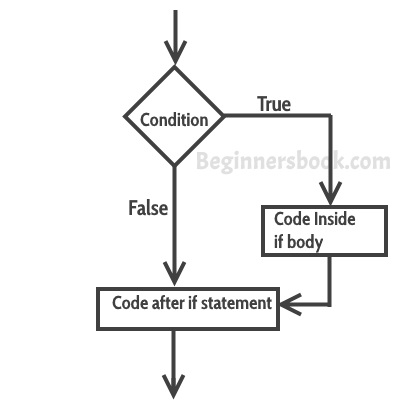## Python – If statement Example

```flag = True
if flag==True:
print("Welcome")
print("To")
print("BeginnersBook.com")```

Output:

```Welcome
To
BeginnersBook.com```

In the above example we are checking the value of flag variable and if the value is True then we are executing few print statements. The important point to note here is that even if we do not compare the value of flag with the ‘True’ and simply put ‘flag’ in place of condition, the code would run just fine so the better way to write the above code would be:

```flag = True
if flag:
print("Welcome")
print("To")
print("BeginnersBook.com")```

By seeing this we can understand how if statement works. The output of the condition would either be true or false. If the outcome of condition is true then the statements inside body of ‘if’ executes, however if the outcome of condition is false then the statements inside ‘if’ are skipped. Lets take another example to understand this:

```flag = False
if flag:
print("You Guys")
print("are")
print("Awesome")```

The output of this code is none, it does not print anything because the outcome of condition is ‘false’.

## Python if example without boolean variables

In the above examples, we have used the boolean variables in place of conditions. However we can use any variables in our conditions. For example:

```num = 100
if num < 200:
print("num is less than 200")```

Output:

```num is less than 200

```

# Python If else Statement Example

In the last tutorial we learned how to use if statements in Python. In this guide, we will learn another control statement ‘if..else’.

We use if statements when we need to execute a certain block of Python code when a particular condition is true. If..else statements are like extension of ‘if’ statements, with the help of if..else we can execute certain statements if condition is true and a different set of statements if condition is false. For example, you want to print ‘even number’ if the number is even and ‘odd number’ if the number is not even, we can accomplish this with the help of if..else statement.

## Python – Syntax of if..else statement

```if condition:
block_of_code_1
else:
block_of_code_2```

block_of_code_1: This would execute if the given condition is true
block_of_code_2: This would execute if the given condition is false

## If..else flow control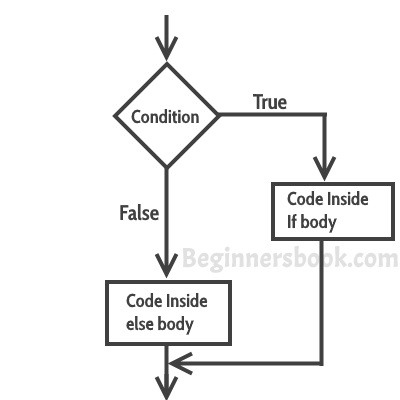## If-else example in Python

```num = 22
if num % 2 == 0:
print("Even Number")
else:
print("Odd Number")```

Output:

`Even Number`

# Python If elif else statement example

In the previous tutorials we have seen if statement and if..else statement. In this tutorial, we will learn if elif else statement in Python. The if..elif..else statement is used when we need to check multiple conditions.

## Syntax of if elif else statement in Python

This way we are checking multiple conditions.

```if condition:
block_of_code_1
elif condition_2:
block_of_code_2
elif condition_3:
block_of_code_3
..
..
..
else:
block_of_code_n```

Notes:
1. There can be multiple ‘elif’ blocks, however there is only ‘else’ block is allowed.
2. Out of all these blocks only one block_of_code gets executed. If the condition is true then the code inside ‘if’ gets executed, if condition is false then the next condition(associated with elif) is evaluated and so on. If none of the conditions is true then the code inside ‘else’ gets executed.

## Python – if..elif..else statement example

In this example, we are checking multiple conditions using if..elif..else statement.

```num = 1122
if 9 < num < 99:
print("Two digit number")
elif 99 < num < 999:
print("Three digit number")
elif 999 < num < 9999:
print("Four digit number")
else:
print("number is <= 9 or >= 9999")```

Output: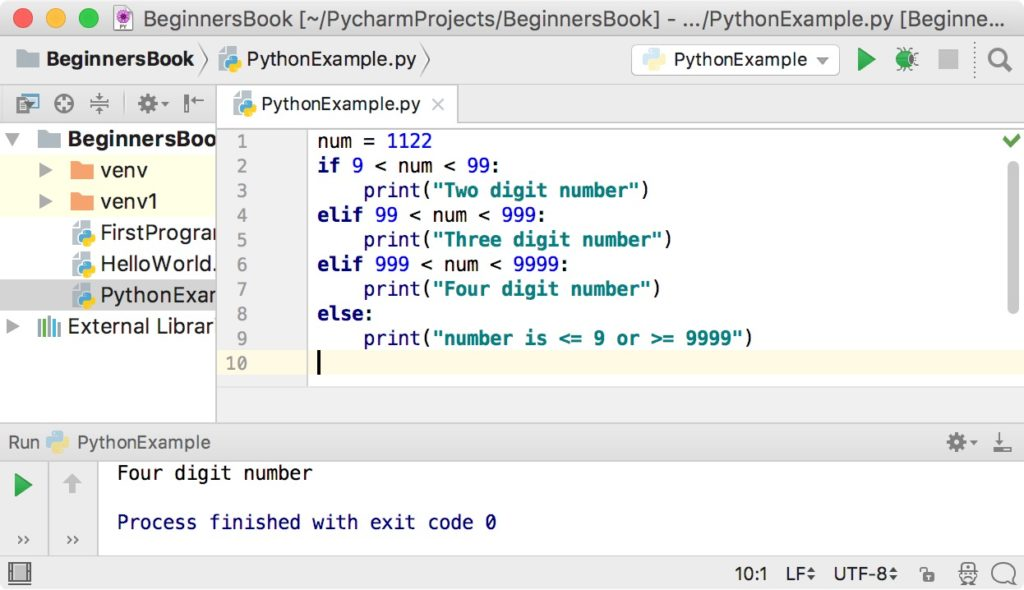` `

# Python Nested If else statement

In the previous tutorials, we have covered the if statementif..else statement and if..elif..else statement. In this tutorial, we will learn the nesting of these control statements.

When there is an if statement (or if..else or if..elif..else) is present inside another if statement (or if..else or if..elif..else) then this is calling the nesting of control statements.

## Nested if..else statement example

Here we have a if statement inside another if..else statement block. Nesting control statements makes us to check multiple conditions.

```num = -99
if num > 0:
print("Positive Number")
else:
print("Negative Number")
#nested if
if -99<=num:
print("Two digit Negative Number")```

Output:

```Negative Number
Two digit Negative Number```

# Python for Loop explained with examples

A loop is a used for iterating over a set of statements repeatedly. In Python we have three types of loops forwhile and do-while. In this guide, we will learn for loop and the other two loops are covered in the separate tutorials.

## Syntax of For loop in Python

```for <variable> in <sequence>:
# body_of_loop that has set of statements
# which requires repeated execution```

Here <variable> is a variable that is used for iterating over a <sequence>. On every iteration it takes the next value from <sequence>  until the end of sequence is reached.

Lets take few examples of for loop to understand the usage.

## Python – For loop example

The following example shows the use of for loop to iterate over a list of numbers. In the body of for loop we are calculating the square of each number present in list and displaying the same.

```# Program to print squares of all numbers present in a list

# List of integer numbers
numbers = [1, 2, 4, 6, 11, 20]

# variable to store the square of each num temporary
sq = 0

# iterating over the given list
for val in numbers:
# calculating square of each number
sq = val * val
# displaying the squares
print(sq)```

Output:

```1
4
16
36
121
400```

## Function range()

In the above example, we have iterated over a list using for loop. However we can also use a range() function in for loop to iterate over numbers defined by range().

range(n): generates a set of whole numbers starting from 0 to (n-1).
For example:
range(8) is equivalent to [0, 1, 2, 3, 4, 5, 6, 7]

range(start, stop): generates a set of whole numbers starting from `start` to `stop-1`.
For example:
range(5, 9) is equivalent to [5, 6, 7, 8]

range(start, stop, step_size): The default step_size is 1 which is why when we didn’t specify the step_size, the numbers generated are having difference of 1. However by specifying step_size we can generate numbers having the difference of step_size.
For example:
range(1, 10, 2) is equivalent to [1, 3, 5, 7, 9]

Lets use the range() function in for loop:

## Python for loop example using range() function

Here we are using range() function to calculate and display the sum of first 5 natural numbers.

```# Program to print the sum of first 5 natural numbers

# variable to store the sum
sum = 0

# iterating over natural numbers using range()
for val in range(1, 6):
# calculating sum
sum = sum + val

# displaying sum of first 5 natural numbers
print(sum)```

Output:

`15`

## For loop with else block

Unlike Java, In Python we can have an optional ‘else’ block associated with the loop. The ‘else’ block executes only when the loop has completed all the iterations. Lets take an example:

```for val in range(5):
print(val)
else:
print("The loop has completed execution")```

Output:

```0
1
2
3
4
The loop has completed execution```

Note: The else block only executes when the loop is finished.

## Nested For loop in Python

When a for loop is present inside another for loop then it is called a nested for loop. Lets take an example of nested for loop.

```for num1 in range(3):
for num2 in range(10, 14):
print(num1, ",", num2)```

Output:

```0 , 10
0 , 11
0 , 12
0 , 13
1 , 10
1 , 11
1 , 12
1 , 13
2 , 10
2 , 11
2 , 12
2 , 13```

# Python While Loop

While loop is used to iterate over a block of code repeatedly until a given condition returns false. In the last tutorial, we have seen for loop in Python, which is also used for the same purpose. The main difference is that we use while loop when we are not certain of the number of times the loop requires execution, on the other hand when we exactly know how many times we need to run the loop, we use for loop.

## Syntax of while loop

```while condition:
#body_of_while```

The body_of_while is set of Python statements which requires repeated execution. These set of statements execute repeatedly until the given condition returns false.

## Flow of while loop

1. First the given condition is checked, if the condition returns false, the loop is terminated and the control jumps to the next statement in the program after the loop.
2. If the condition returns true, the set of statements inside loop are executed and then the control jumps to the beginning of the loop for next iteration.

These two steps happen repeatedly as long as the condition specified in while loop remains true.

## Python – While loop example

Here is an example of while loop. In this example, we have a variable `num` and we are displaying the value of `num` in a loop, the loop has a increment operation where we are increasing the value of `num`. This is very important step, the while loop must have a increment or decrement operation, else the loop will run indefinitely, we will cover this later in infinite while loop.

```num = 1
# loop will repeat itself as long as
# num < 10 remains true
while num < 10:
print(num)
#incrementing the value of num
num = num + 3```

Output:

```1
4
7```

## Infinite while loop

Example 1:
This will print the word ‘hello’ indefinitely because the condition will always be true.

```while True:
print("hello")```

Example 2:

```num = 1
while num<5:
print(num)```

This will print ‘1’ indefinitely because inside loop we are not updating the value of num, so the value of num will always remain 1 and the condition num < 5 will always return true.

## Nested while loop in Python

When a while loop is present inside another while loop then it is called nested while loop. Lets take an example to understand this concept.

```i = 1
j = 5
while i < 4:
while j < 8:
print(i, ",", j)
j = j + 1
i = i + 1```

Output:

```1 , 5
2 , 6
3 , 7```

## Python – while loop with else block

We can have a ‘else’ block associated with while loop. The ‘else’ block is optional. It executes only after the loop finished execution.

```num = 10
while num > 6:
print(num)
num = num-1
else:
print("loop is finished")```

Output:

```10
9
8
7
loop is finished```

# Python break Statement

The break statement is used to terminate the loop when a certain condition is met. We already learned in previous tutorials (for loop and while loop) that a loop is used to iterate a set of statements repeatedly as long as the loop condition returns true. The break statement is generally used inside a loop along with a if statement so that when a particular condition (defined in if statement) returns true, the break statement is encountered and the loop terminates.

For example, lets say we are searching an element in a list, so for that we are running a loop starting from the first element of the list to the last element of the list. Using break statement, we can terminate the loop as soon as the element is found because why run the loop unnecessary till the end of list when our element is found. We can achieve this with the help of break statement (we will see this example programmatically in the example section below).

## Syntax of break statement in Python

The syntax of break statement in Python is similar to what we have seen in Java.

`break`

## Flow diagram of break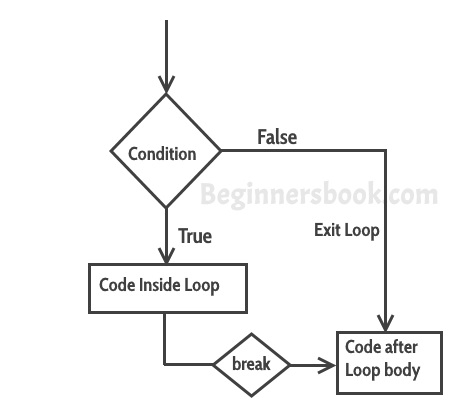## Example of break statement

In this example, we are searching a number ’88’ in the given list of numbers. The requirement is to display all the numbers till the number ’88’ is found and when it is found, terminate the loop and do not display the rest of the numbers.

```# program to display all the elements before number 88
for num in [11, 9, 88, 10, 90, 3, 19]:
print(num)
if(num==88):
print("The number 88 is found")
print("Terminating the loop")
break```

Output:

```11
9
88
The number 88 is found
Terminating the loop```

Note: You would always want to use the break statement with a if statement so that only when the condition associated with ‘if’ is true then only break is encountered. If you do not use it with ‘if’ statement then the break statement would be encountered in the first iteration of loop and the loop would always terminate on the first iteration.

# Python Continue Statement

The continue statement is used inside a loop to skip the rest of the statements in the body of loop for the current iteration and jump to the beginning of the loop for next iteration. The break and continue statements are used to alter the flow of loop, break terminates the loop when a condition is met and continue skip the current iteration.

## Syntax of continue statement in Python

The syntax of continue statement in Python is similar to what we have seen in Java(except the semicolon)

`continue`

## Flow diagram of continue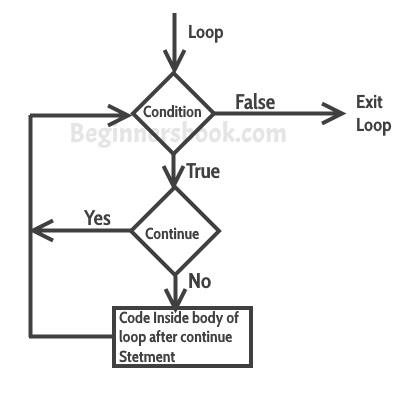## Example of continue statement

Lets say we have a list of numbers and we want to print only the odd numbers out of that list. We can do this by using continue statement.
We are skipping the print statement inside loop by using continue statement when the number is even, this way all the even numbers are skipped and the print statement executed for all the odd numbers.

# program to display only odd numbers
```for num in [20, 11, 9, 66, 4, 89, 44]:
# Skipping the iteration when number is even
if num%2 == 0:
continue
# This statement will be skipped for all even numbers
print(num)```

Output:

```11
9
89```

# Python pass Statement

The pass statement acts as a placeholder and usually used when there is no need of code but a statement is still required to make a code syntactically correct. For example we want to declare a function in our code but we want to implement that function in future, which means we are not yet ready to write the body of the function. In this case we cannot leave the body of function empty as this would raise error because it is syntactically incorrect, in such cases we can use pass statement which does nothing but makes the code syntactically correct.

## Pass statement vs comment

You may be wondering that a python comment works similar to the pass statement as it does nothing so we can use comment in place of pass statement. Well, it is not the case, a comment is not a placeholder and it is completely ignored by the Python interpreter while on the other hand `pass` is not ignored by interpreter, it says the interpreter to do nothing.

## Python pass statement example

If the number is even we are doing nothing and if it is odd then we are displaying the number.

```for num in [20, 11, 9, 66, 4, 89, 44]:
if num%2 == 0:
pass
else:
print(num)```

Output:

11
```9
89```

### Other examples:

A function that does nothing(yet), may be implemented in future.

`def f(arg): pass    # a function that does nothing (yet)`

A class that does not have any methods(yet), may have methods in future implementation.

`class C: pass       # a class with no methods (yet)`

#### Reference

Python docs – pass statement

## Python Functions

#### In this guide, we will learn about functions in Python. A function is a block of code that contains one or more Python statements and used for performing a specific task.

Why use function in Python?
As I mentioned above, a function is a block of code that performs a specific task. Lets discuss what we can achieve in Python by using functions in our code:
1. Code re-usability: Lets say we are writing an application in Python where we need to perform a specific task in several places of our code, assume that we need to write 10 lines of code to do that specific task. It would be better to write those 10 lines of code in a function and just call the function wherever needed, because writing those 10 lines every time you perform that task is tedious, it would make your code lengthy, less-readable and increase the chances of human errors.

2. Improves Readability: By using functions for frequent tasks you make your code structured and readable. It would be easier for anyone to look at the code and be able to understand the flow and purpose of the code.

3. Avoid redundancy: When you no longer repeat the same lines of code throughout the code and use functions in places of those, you actually avoiding the redundancy that you may have created by not using functions.

Syntax of functions in Python
Function declaration:

def function_name(function_parameters):
function_body # Set of Python statements
return # optional return statement
Calling the function:

# when function doesn’t return anything
function_name(parameters)
OR

# when function returns something
# variable is to store the returned value
variable = function_name(parameters)
Python Function example
Here we have a function add() that adds two numbers passed to it as parameters. Later after function declaration we are calling the function twice in our program to perform the addition.

return num1 + num2

print(sum1)
print(sum2)
Output:

300
17
Default arguments in Function
Now that we know how to declare and call a function, lets see how can we use the default arguments. By using default arguments we can avoid the errors that may arise while calling a function without passing all the parameters. Lets take an example to understand this:

In this example we have provided the default argument for the second parameter, this default argument would be used when we do not provide the second parameter while calling this function.

# default argument for second parameter
return num1 + num2

sum2 = add(8) # used default argument for second param
sum3 = add(100) # used default argument for second param
print(sum1)
print(sum2)
print(sum3)
Output:

300
9
101
Types of functions
There are two types of functions in Python:
1. Built-in functions: These functions are predefined in Python and we need not to declare these functions before calling them. We can freely invoke them as and when needed.
2. User defined functions: The functions which we create in our code are user-defined functions. The add() function that we have created in above examples is a user-defined function.

We will cover more about these function types in the separate guides.

# Python Recursion

A function is said to be a recursive if it calls itself. For example, lets say we have a function `abc()` and in the body of `abc()` there is a call to the `abc()`.

## Python example of Recursion

In this example we are defining a user-defined function `factorial()`. This function finds the factorial of a number by calling itself repeatedly until the base case(We will discuss more about base case later, after this example) is reached.

```# Example of recursion in Python to
# find the factorial of a given number

def factorial(num):
"""This function calls itself to find
the factorial of a number"""

if num == 1:
return 1
else:
return (num * factorial(num - 1))

num = 5
print("Factorial of", num, "is: ", factorial(num))```

Output:

`Factorial of 5 is:  120`

Lets see what happens in the above example:

factorial(5) returns 5 * factorial(51)
```    i.e. 5 * factorial(4)
|__5*4*factorial(3)
|__5*4*3*factorial(2)
|__5*4*3*2*factorial(1)```

Note: factorial(1) is a base case for which we already know the value of factorial. The base case is defined in the body of function with this code:

```if num == 1:
return 1```

## What is a base case in recursion

When working with recursion, we should define a base case for which we already know the answer. In the above example we are finding factorial of an integer number and we already know that the factorial of 1 is 1 so this is our base case.

Each successive recursive call to the function should bring it closer to the base case, which is exactly what we are doing in above example.

We use base case in recursive function so that the function stops calling itself when the base case is reached. Without the base case, the function would keep calling itself indefinitely.

## Why use recursion in programming?

We use recursion to break a big problem in small problems and those small problems into further smaller problems and so on. At the end the solutions of all the smaller subproblems are collectively helps in finding the solution of the big main problem.

Recursion makes our program:
1. Easier to write.
3. Reduce the lines of code – It takes less lines of code to solve a problem using recursion.

1. Not all problems can be solved using recursion.
2. If you don’t define the base case then the code would run indefinitely.
3. Debugging is difficult in recursive functions as the function is calling itself in a loop and it is hard to understand which call is causing the issue.
4. Memory overhead – Call to the recursive function is not memory efficient.

# Python Numbers

In this guide, we will see how to work with numbers in Python. Python supports integers, floats and complex numbers.

An integer is a number without decimal point for example 5, 6, 10 etc.

float is a number with decimal point for example 6.7, 6.0, 10.99 etc.

complex number has a real and imaginary part for example 7+8j, 8+11j etc.

## Example: Numbers in Python

```# Python program to display numbers of
# different data types

# int
num1 = 10
num2 = 100
print(num1+num2)

# float
a = 10.5
b = 8.9
print(a-b)

# complex numbers
x = 3 + 4j
y = 9 + 8j
print(y-x)```

Output:

```110
1.5999999999999996
(6+4j)```

## Python example to find the class(data type) of a number

We can use the `type()` function to find out the class of a number. An integer number belongs to `int` class, a float number belongs to `float` class and a complex number belongs to `complex` class.

```# program to find the class of a number

# int
num = 100
print("type of num: ",type(num))

# float
num2 = 10.99
print("type of num2: ",type(num2))

# complex numbers
num3 = 3 + 4j
print("type of num3: ",type(num3))```

Output: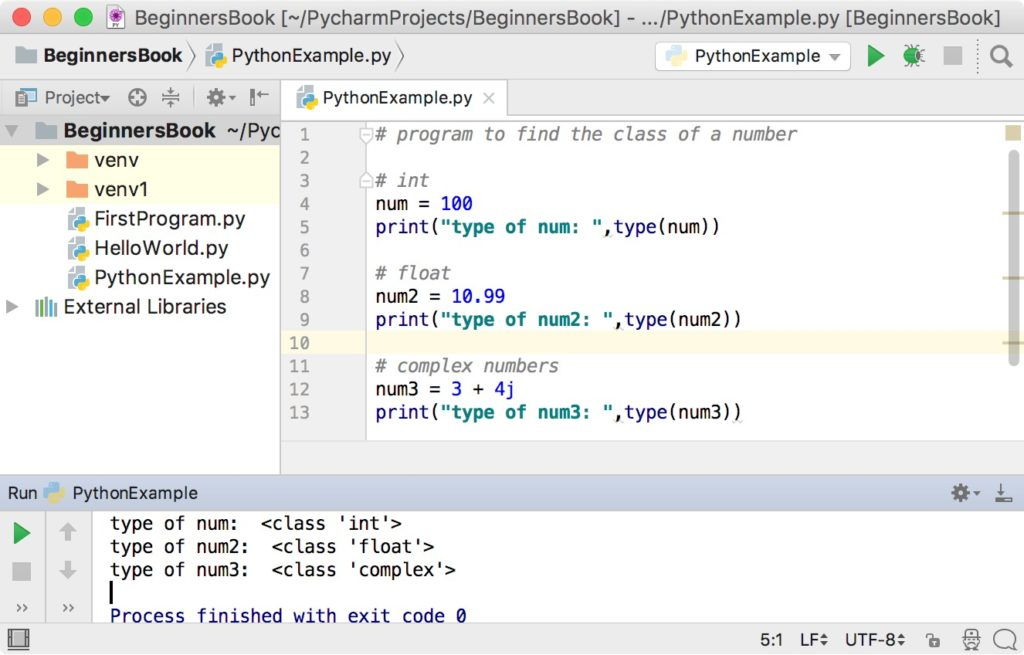## The isinstance() function

The isinstance() functions checks whether a number belongs to a particular class and returns true or false based on the result.
For example:
`isinstance(num, int)` will return true if the number `num` is an integer number.
`isinstance(num, int)` will return false if the number `num` is not an integer number.

### Example of isinstance() function

```num = 100
# true because num is an integer
print(isinstance(num, int))

# false because num is not a float
print(isinstance(num, float))

# false because num is not a complex number
print(isinstance(num, complex))```

Output:

```True
False
False```
` `